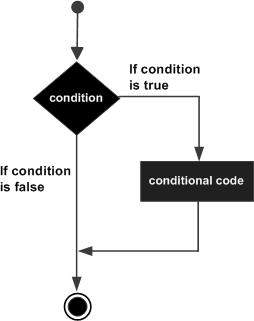# VBA - If Statement

An If statement consists of a Boolean expression followed by one or more statements. If the condition is said to be True, the statements under If condition(s) are executed. If the condition is said to be False, the statements after the If loop are executed.

## Syntax

Following is the syntax of an If statement in VBScript.

```If(boolean_expression) Then
Statement 1
.....
.....
Statement n
End If
```

## Flow Diagram### Example

For demo purpose, let us find the biggest between the two numbers of an Excel with the help of a function.

```Private Sub if_demo_Click()
Dim x As Integer
Dim y As Integer

x = 234
y = 32

If x > y Then
MsgBox "X is Greater than Y"
End If
End Sub
```

When the above code is executed, it produces the following result.

```X is Greater than Y
```
vba_decisions.htm